# INTRODUCTION TO R Compute Fest 2012 Alex Storer

• Slides: 45
Download presentationINTRODUCTION TO R Compute. Fest 2012 Alex Storer Institute for Quantitative Social Science at Harvard [email protected] harvard. edu http: //www. people. fas. harvard. edu/~astorer/computefest 2012/1/20/12 Introduction to R (Alex Storer, IQSS) Introduction • Research Technology Consulting at IQSS • Dataverse • Research Computing Environment • Open. Scholar • Packages for computational text analysis, multiple imputation, and statistics modeling in R • Trainings on R, Stata, SAS http: //iq. harvard. edu 2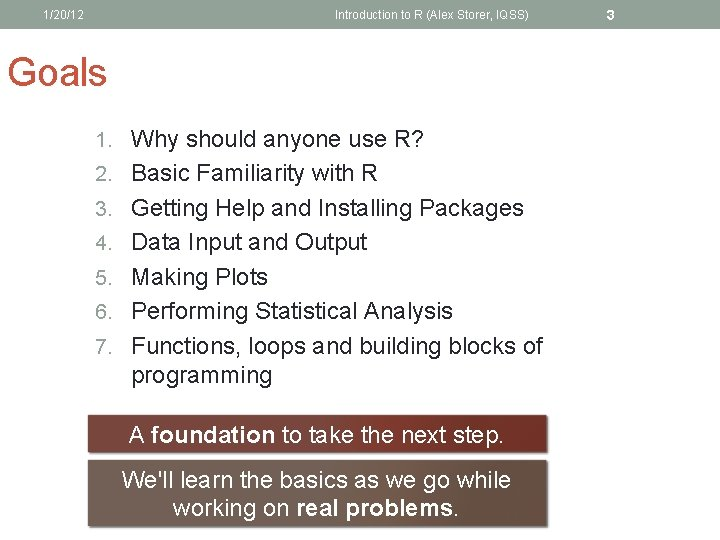1/20/12 Introduction to R (Alex Storer, IQSS) Goals 1. Why should anyone use R? 2. Basic Familiarity with R 3. Getting Help and Installing Packages 4. Data Input and Output 5. Making Plots 6. Performing Statistical Analysis 7. Functions, loops and building blocks of programming A foundation to take the next step. We'll learn the basics as we go while working on real problems. 31/20/12 Introduction to R (Alex Storer, IQSS) 4 Why should I use R? “I already know Matlab. Why would I want to use R? ” 1. Matlab is expensive. 2. R is open-source and community developed. 3. R is platform independent (and can even be run online) 4. Beautiful graphics with clear syntax 5. The most actively developed cutting-edge statistics applications can be installed from inside R!1/20/12 Introduction to R (Alex Storer, IQSS) 5 Why should I use R? “I already know Python. Why would I ever want to learn a new language that already does a subset of what Python already does? ” 1. R is designed to do data-processing and statistical analysis, and it makes it easy. 2. More support in R (e. g. , the Use R! series) 3. More active development in R for data analysis 4. Who are you sharing your code with?1/20/12 Starting R Introduction to R (Alex Storer, IQSS) 61/20/12 7 Introduction to R (Alex Storer, IQSS) Syntax Notes R Matlab Python x <- seq(1, 10) # or x <- 1: 10 # or x = 1: 10 %a less flexible %version of linspace x = range(1, 11) # indices start at 0 for (i in x) {print("hello")} for (i = x) disp("hello") end for i in x: print("hello") foo. bar <- 10 foo. bar = 10 > foo. bar  10 > foo. bar foo = bar: 10 Name. Error: name 'foo' is not defined1/20/12 Introduction to R (Alex Storer, IQSS) 8 Vectors in R > x <- c(1, 1, 2, 3, 5, 8, 13, 21) > length(x)  8 > x  5 The c() command concatenates items into a vector of the same type! Note that R counts from 1.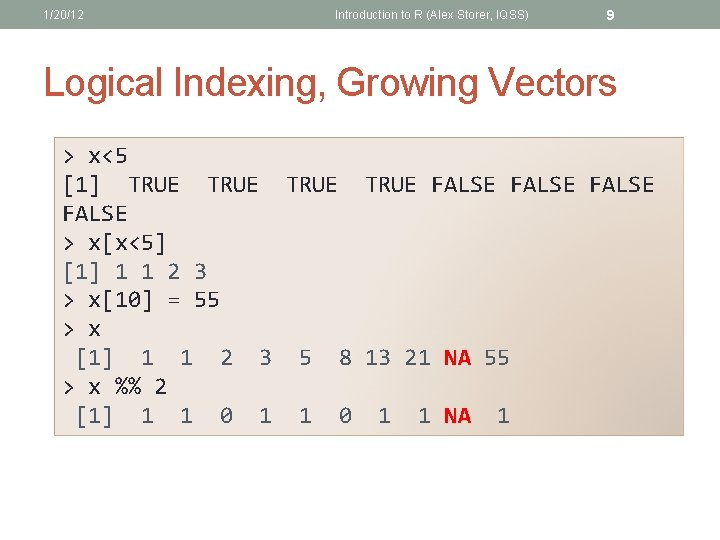1/20/12 Introduction to R (Alex Storer, IQSS) 9 Logical Indexing, Growing Vectors > x<5  TRUE FALSE > x[x<5]  1 1 2 3 > x = 55 > x  1 1 2 3 5 8 13 21 NA 55 > x %% 2  1 1 0 1 1 NA 1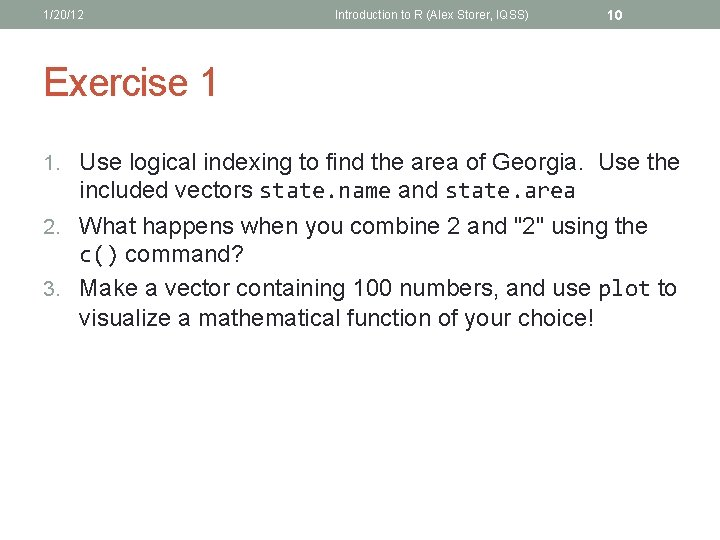1/20/12 Introduction to R (Alex Storer, IQSS) 10 Exercise 1 1. Use logical indexing to find the area of Georgia. Use the included vectors state. name and state. area 2. What happens when you combine 2 and "2" using the c() command? 3. Make a vector containing 100 numbers, and use plot to visualize a mathematical function of your choice!1/20/12 Introduction to R (Alex Storer, IQSS) 11 One more function… > help("foo") > ? foo Basics Simple Description Function call Arguments Details How the function works The author Relevant Notes See Also Other relevant functions Examples Several sample calls Return Values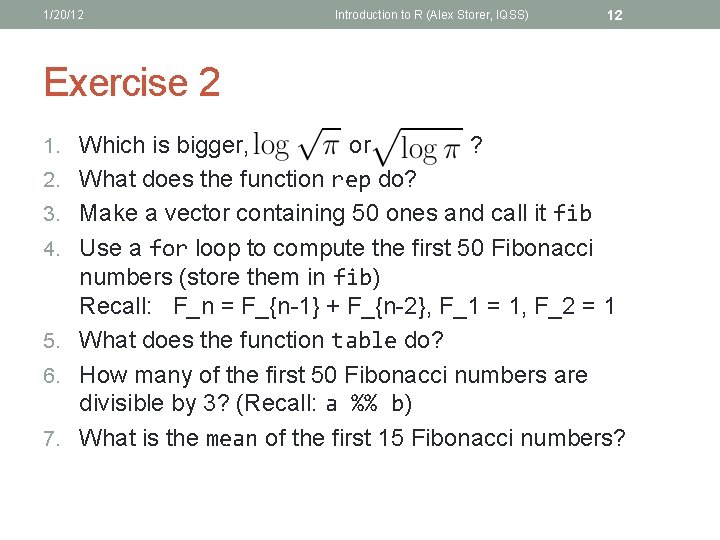1/20/12 Introduction to R (Alex Storer, IQSS) 12 Exercise 2 1. Which is bigger, 2. 3. 4. 5. 6. 7. or ? What does the function rep do? Make a vector containing 50 ones and call it fib Use a for loop to compute the first 50 Fibonacci numbers (store them in fib) Recall: F_n = F_{n-1} + F_{n-2}, F_1 = 1, F_2 = 1 What does the function table do? How many of the first 50 Fibonacci numbers are divisible by 3? (Recall: a %% b) What is the mean of the first 15 Fibonacci numbers?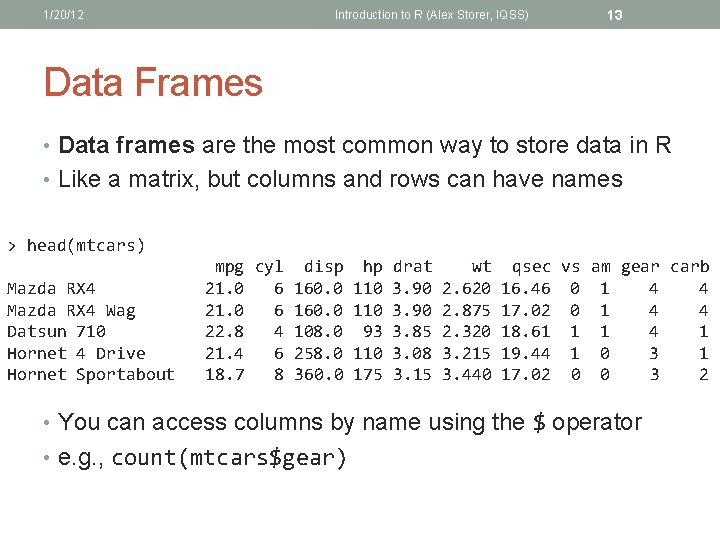1/20/12 Introduction to R (Alex Storer, IQSS) 13 Data Frames • Data frames are the most common way to store data in R • Like a matrix, but columns and rows can have names > head(mtcars) Mazda RX 4 Wag Datsun 710 Hornet 4 Drive Hornet Sportabout mpg cyl disp hp drat wt qsec vs am gear carb 21. 0 6 160. 0 110 3. 90 2. 620 16. 46 0 1 4 4 21. 0 6 160. 0 110 3. 90 2. 875 17. 02 0 1 4 4 22. 8 4 108. 0 93 3. 85 2. 320 18. 61 1 1 4 1 21. 4 6 258. 0 110 3. 08 3. 215 19. 44 1 0 3 1 18. 7 8 360. 0 175 3. 15 3. 440 17. 02 0 0 3 2 • You can access columns by name using the \$ operator • e. g. , count(mtcars\$gear)1/20/12 Introduction to R (Alex Storer, IQSS) 14 Data Frames: viewing and manipulating > > > summary(mtcars) write. csv(mtcars, '/tmp/blah. csv') mtcars\$logmpg <- log(mtcars\$mpg) rownames(mtcars) colnames(mtcars)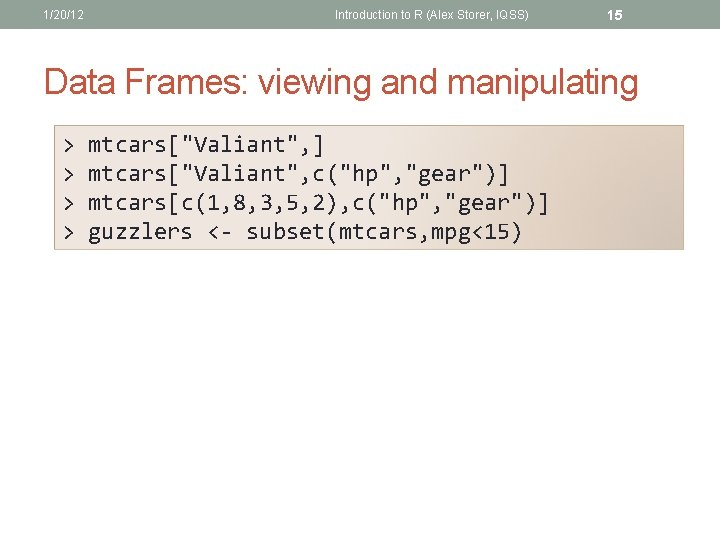1/20/12 Introduction to R (Alex Storer, IQSS) 15 Data Frames: viewing and manipulating > > mtcars["Valiant", ] mtcars["Valiant", c("hp", "gear")] mtcars[c(1, 8, 3, 5, 2), c("hp", "gear")] guzzlers <- subset(mtcars, mpg<15)1/20/12 Introduction to R (Alex Storer, IQSS) 16 Exercise 2 1. What is the median mpg for cars with horsepower ("hp") less than 100? 2. Use the order command to print the state. name sorted by state. area 3. Use the order command write. csv to save the mtcars dataset sorted by horsepower 4. What is the name of the car with the most horsepower out of all cars with 4 cylinders ("cyl")?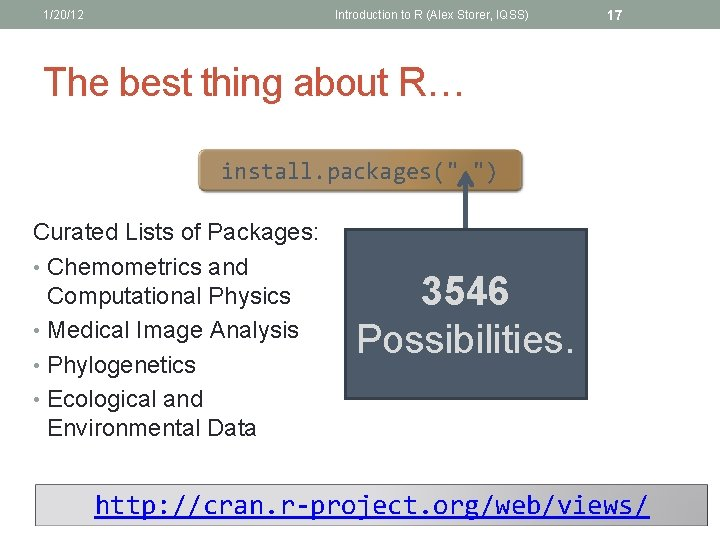1/20/12 Introduction to R (Alex Storer, IQSS) 17 The best thing about R… install. packages(" ") Curated Lists of Packages: • Chemometrics and Computational Physics • Medical Image Analysis • Phylogenetics • Ecological and Environmental Data 3546 Possibilities. http: //cran. r-project. org/web/views/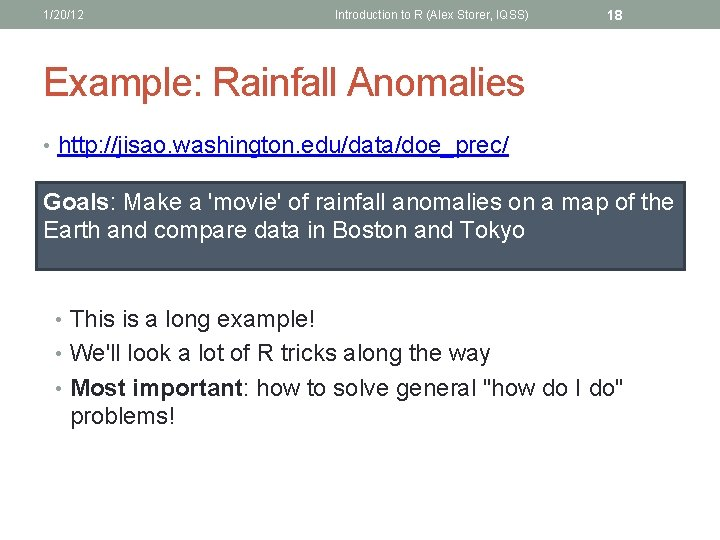1/20/12 Introduction to R (Alex Storer, IQSS) 18 Example: Rainfall Anomalies • http: //jisao. washington. edu/data/doe_prec/ Goals: Make a 'movie' of rainfall anomalies on a map of the Earth and compare data in Boston and Tokyo • This is a long example! • We'll look a lot of R tricks along the way • Most important: how to solve general "how do I do" problems!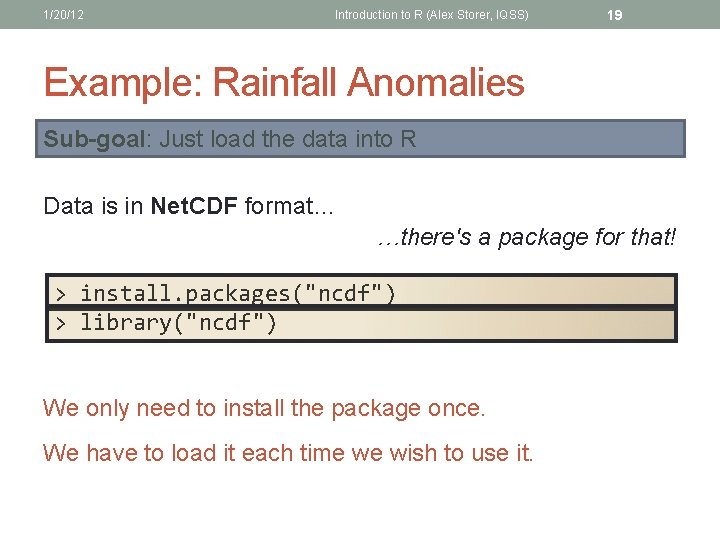1/20/12 Introduction to R (Alex Storer, IQSS) 19 Example: Rainfall Anomalies Sub-goal: Just load the data into R Data is in Net. CDF format… …there's a package for that! > install. packages("ncdf") > library("ncdf") We only need to install the package once. We have to load it each time we wish to use it.1/20/12 Introduction to R (Alex Storer, IQSS) 20 Example: Rainfall Anomalies Sub-goal: Just load the data into R Let's just get the data! > sfile <"http: //jisao. washington. edu/data/doe_prec/pre cipanom 18511989. nc" > download. file(sfile, "/tmp/recip. nc") > dat <- open. ncdf("/tmp/recip. nc") > datthe use of '/' (this can trip you up in Windows!) Notice   "file /tmp/recip. nc has 3 dimensions: " "lat Size: 44" "lon Size: 72" "time Size: 556" "------------" "file /tmp/recip. nc has 1 variables: " "short data[lon, lat, time] Longname: djf, mam, jja, son mm. Missval: 32767"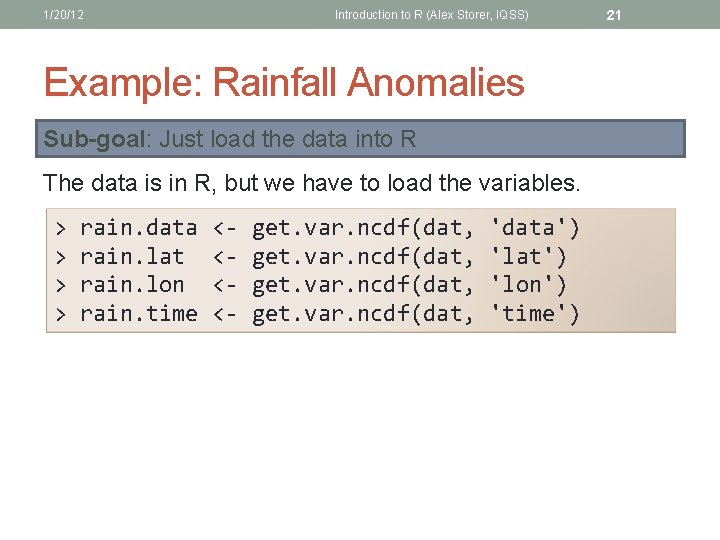1/20/12 Introduction to R (Alex Storer, IQSS) Example: Rainfall Anomalies Sub-goal: Just load the data into R The data is in R, but we have to load the variables. > > rain. data rain. lat rain. lon rain. time <<<<- get. var. ncdf(dat, 'data') 'lat') 'lon') 'time') 21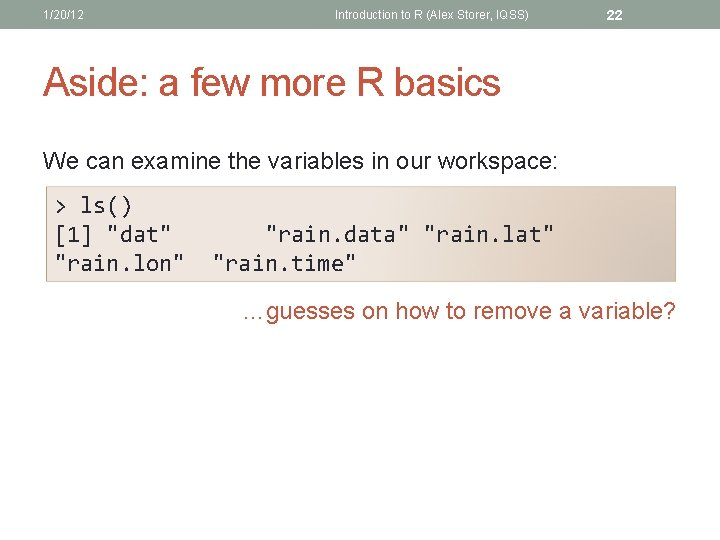1/20/12 Introduction to R (Alex Storer, IQSS) 22 Aside: a few more R basics We can examine the variables in our workspace: > ls()  "dat" "rain. lon" "rain. data" "rain. lat" "rain. time" …guesses on how to remove a variable?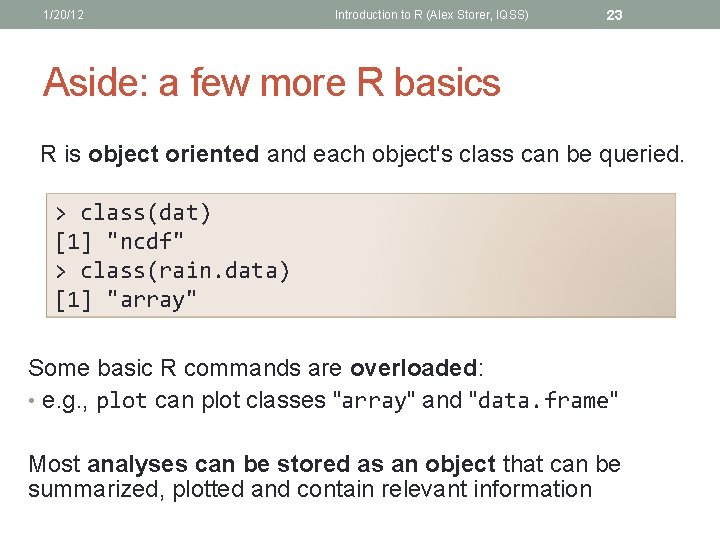1/20/12 Introduction to R (Alex Storer, IQSS) 23 Aside: a few more R basics R is object oriented and each object's class can be queried. > class(dat)  "ncdf" > class(rain. data)  "array" Some basic R commands are overloaded: • e. g. , plot can plot classes "array" and "data. frame" Most analyses can be stored as an object that can be summarized, plotted and contain relevant information1/20/12 Introduction to R (Alex Storer, IQSS) 24 Exercises 3 1. Use the diff command to investigate the spacing of 2. 3. 4. 5. the data points in time Make a new variable called rain. lat. inc that contains the latitude values in increasing order. (Hint: can you reverse the array? Use the seq function to index the array from the end to the beginning. ) Use the ls command to verify that this variable now exists and check its object type using class. Make an array containing all points at time 300. Bonus! Figure out how to write a function that reverses an array. (Try ? function)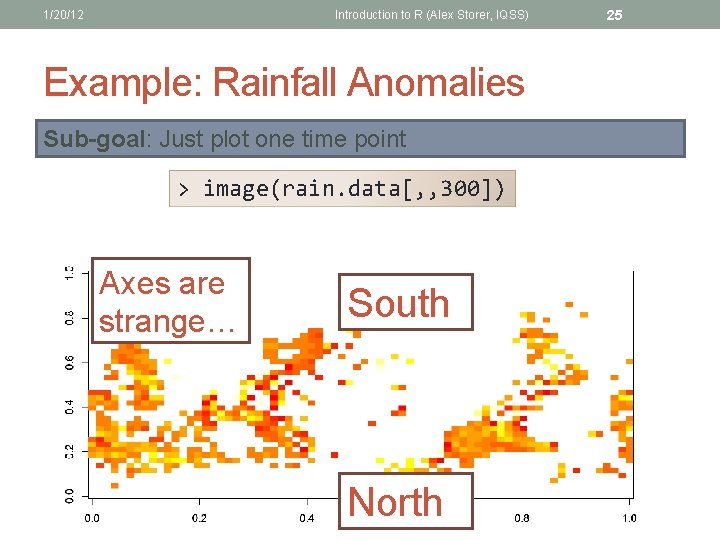1/20/12 Introduction to R (Alex Storer, IQSS) Example: Rainfall Anomalies Sub-goal: Just plot one time point > image(rain. data[, , 300]) Axes are strange… South North 25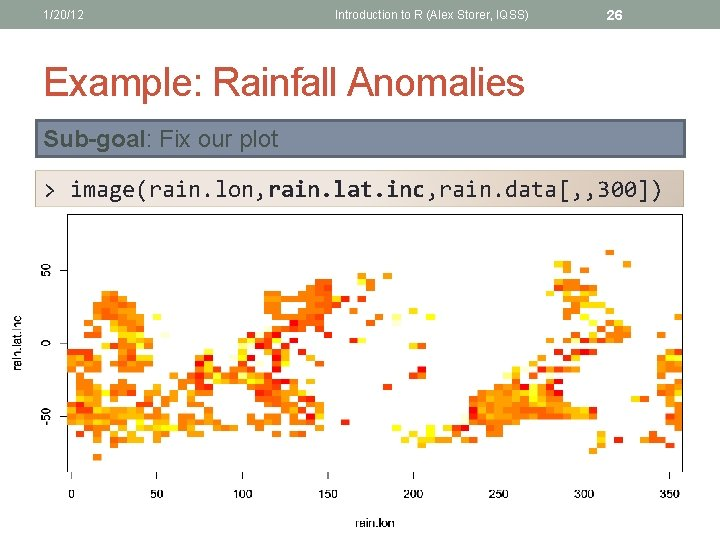1/20/12 Introduction to R (Alex Storer, IQSS) 26 Example: Rainfall Anomalies Sub-goal: Fix our plot > image(rain. lon, rain. lat. inc, rain. data[, , 300])1/20/12 Introduction to R (Alex Storer, IQSS) 27 Example: Rainfall Anomalies Sub-goal: Fix our plot revinds <- length(rain. lat): 1 image(x=rain. lon, y=rain. lat. inc, rain. data[, revinds, 300], xlab="Longitude", ylab="Latitude", main="Rainfall Anomalies") Map?1/20/12 Introduction to R (Alex Storer, IQSS) Package: maps > > install. packages("maps") library("maps") map("world 2") 28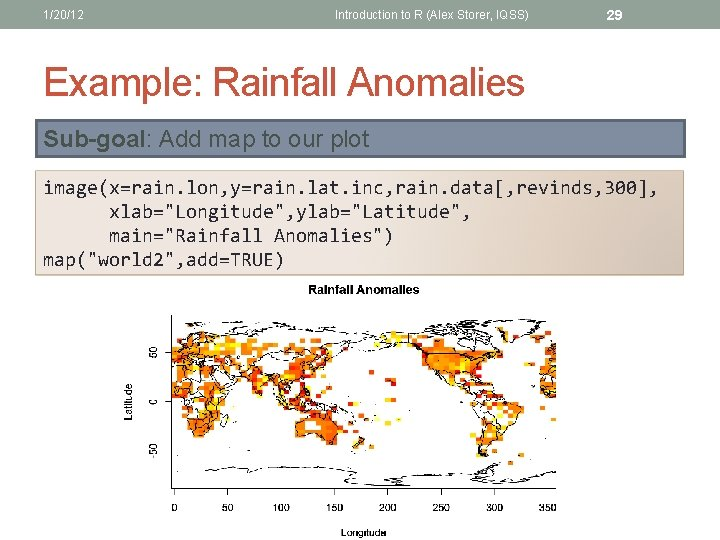1/20/12 Introduction to R (Alex Storer, IQSS) 29 Example: Rainfall Anomalies Sub-goal: Add map to our plot image(x=rain. lon, y=rain. lat. inc, rain. data[, revinds, 300], xlab="Longitude", ylab="Latitude", main="Rainfall Anomalies") map("world 2", add=TRUE)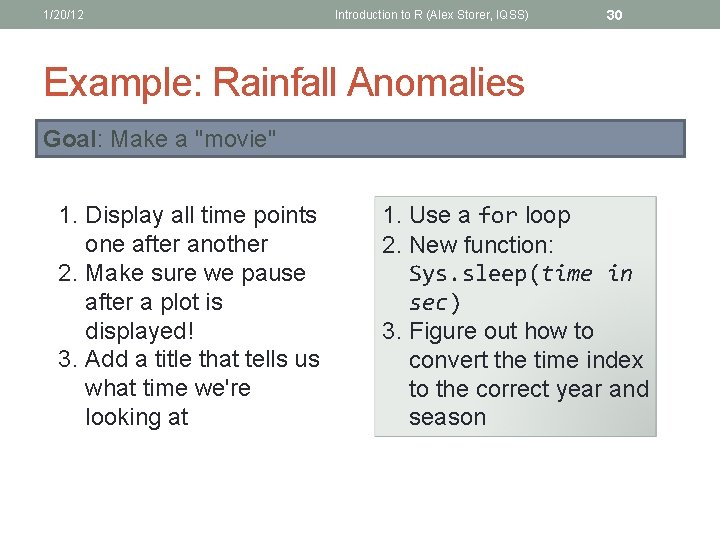1/20/12 Introduction to R (Alex Storer, IQSS) 30 Example: Rainfall Anomalies Goal: Make a "movie" 1. Display all time points one after another 2. Make sure we pause after a plot is displayed! 3. Add a title that tells us what time we're looking at 1. Use a for loop 2. New function: Sys. sleep(time in sec) 3. Figure out how to convert the time index to the correct year and season1/20/12 Introduction to R (Alex Storer, IQSS) 31 Example: Rainfall Anomalies Goal: Make a "movie" seasons = c("Winter", "Spring", "Summer", "Fall") year. start = 1855 for (i in 1: length(rain. time)) { Sys. sleep(0. 05) this. season <- seasons[(i %% 4) + 1] this. year <- floor(i/4)+year. start image(x=rain. lon, y=rain. lat. inc, rain. data[, revinds, i], xlab="Longitude", ylab="Latitude", main=paste('Rainfall Anomalies', 'n', this. year, ': ', this. season)) map("world 2", add=TRUE) }1/20/12 Introduction to R (Alex Storer, IQSS) 32 Example: Rainfall Anomalies Goal: Compare Anomalies in Boston and Tokyo 1. Find the lat/lon values of Boston and Tokyo 2. Find the indices for those values 3. Make a time-series for each location 1. Google it! 2. The which function 3. R provides a ts object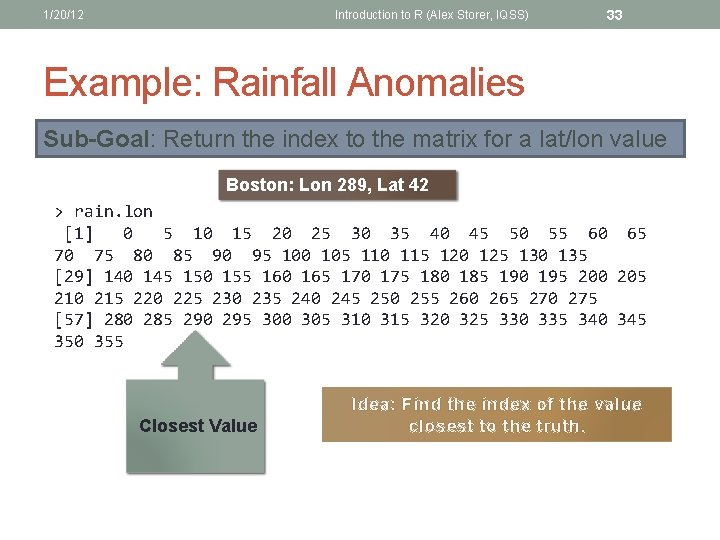1/20/12 Introduction to R (Alex Storer, IQSS) 33 Example: Rainfall Anomalies Sub-Goal: Return the index to the matrix for a lat/lon value Boston: Lon 289, Lat 42 > rain. lon  0 5 10 15 20 25 30 35 40 45 50 55 60 65 70 75 80 85 90 95 100 105 110 115 120 125 130 135  140 145 150 155 160 165 170 175 180 185 190 195 200 205 210 215 220 225 230 235 240 245 250 255 260 265 270 275  280 285 290 295 300 305 310 315 320 325 330 335 340 345 350 355 Closest Value Idea: Find the index of the value closest to the truth.1/20/12 Introduction to R (Alex Storer, IQSS) 34 Example: Rainfall Anomalies Sub-Goal: Find the index of a given lat/lon value boston. lon <- 289; boston. lat <- 42 tokyo. lon <- 139; tokyo. lat <- 35 bos. lon. dist <- abs(boston. lon-rain. lon) bos. lon. ind = which(bos. lon. dist==min(bos. lon. dist)) Get the index of the value that equals the minimum distance bos. lat. ind = which. min(abs(boston. lat-rain. lat)) tok. lon. ind = which. min(abs(tokyo. lon-rain. lon)) tok. lat. ind = which. min(abs(tokyo. lat-rain. lat))1/20/12 Introduction to R (Alex Storer, IQSS) 35 Exercise 4 1. Pick a city and find its indices in the array 2. Use summary to investigate the range of the data in your city 3. Use hist to make a histogram of your city's rainfall data 4. Does this data look normal? Use qqplot to investigate. 5. Figure out how to run a Shapiro-Wilk test and interpret its results1/20/12 Introduction to R (Alex Storer, IQSS) 36 Example: Rainfall Anomalies Sub-Goal: Make a time-series object for Boston and Tokyo bos. ts <- ts(rain. data[bos. lon. ind, bos. lat. ind, ], start=year. start, frequency=4) tok. ts <- ts(rain. dat[tok. lon. ind, tok. lat. ind, ], start=year. start, frequency=4)1/20/12 Introduction to R (Alex Storer, IQSS) Time Series Analysis First Step: Plot your data! plot(bos. ts, type='l', ylab="Anomalies") lines(tok. ts, col="red") 37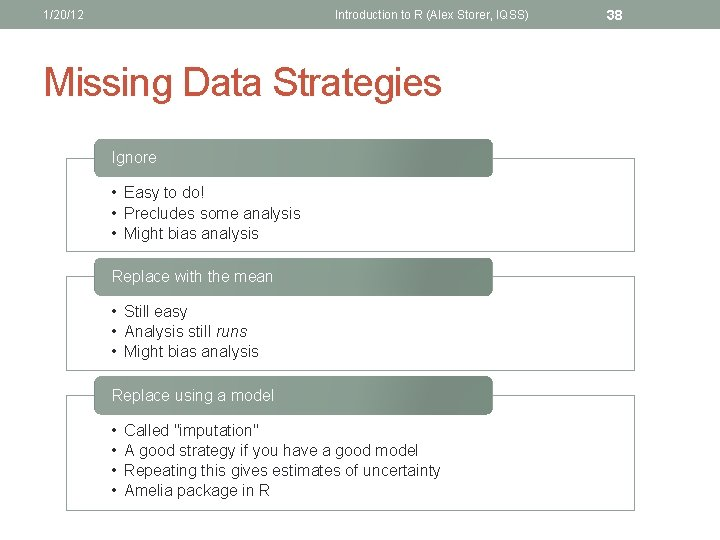1/20/12 Introduction to R (Alex Storer, IQSS) Missing Data Strategies Ignore • Easy to do! • Precludes some analysis • Might bias analysis Replace with the mean • Still easy • Analysis still runs • Might bias analysis Replace using a model • • Called "imputation" A good strategy if you have a good model Repeating this gives estimates of uncertainty Amelia package in R 381/20/12 Introduction to R (Alex Storer, IQSS) 39 Missing Data Strategies • Probably makes sense to ignore • Many procedures in R will crash by default with NA values!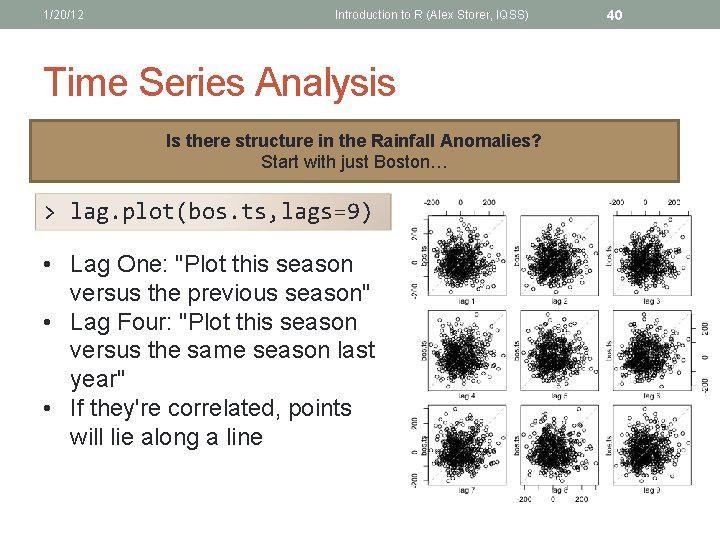1/20/12 Introduction to R (Alex Storer, IQSS) Time Series Analysis Is there structure in the Rainfall Anomalies? Start with just Boston… > lag. plot(bos. ts, lags=9) • Lag One: "Plot this season versus the previous season" • Lag Four: "Plot this season versus the same season last year" • If they're correlated, points will lie along a line 401/20/12 41 Introduction to R (Alex Storer, IQSS) Time Series Analysis Is there structure in the Rainfall Anomalies? Start with just Boston… > acf(bos. ts) • Look at the correlation coefficients all at once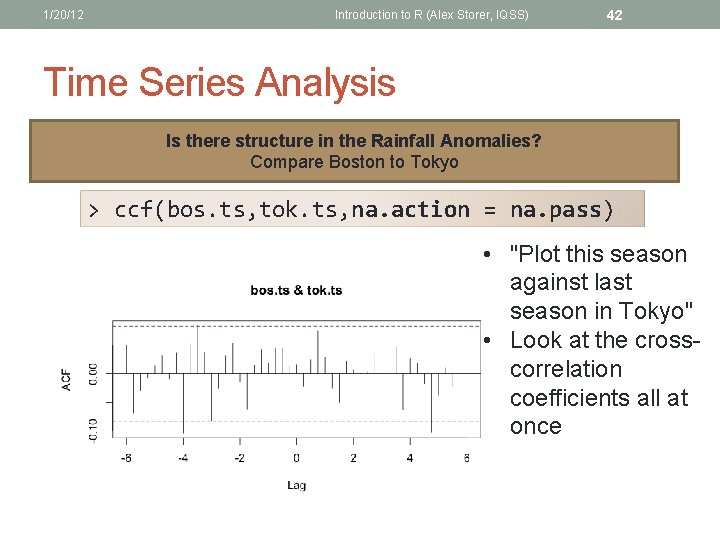1/20/12 Introduction to R (Alex Storer, IQSS) 42 Time Series Analysis Is there structure in the Rainfall Anomalies? Compare Boston to Tokyo > ccf(bos. ts, tok. ts, na. action = na. pass) • "Plot this season against last season in Tokyo" • Look at the crosscorrelation coefficients all at onceIQSS, Harvard University More Time Series Data > data(sunspots); plot(sunspots) 43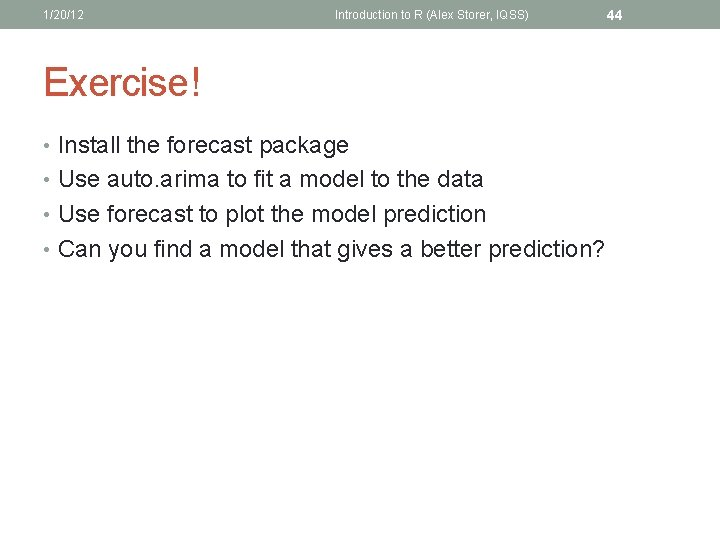1/20/12 Introduction to R (Alex Storer, IQSS) Exercise! • Install the forecast package • Use auto. arima to fit a model to the data • Use forecast to plot the model prediction • Can you find a model that gives a better prediction? 441/20/12 Introduction to R (Alex Storer, IQSS) Thank You! • Doing research in social science? [email protected] hmdc. harvard. edu Feedback: bit. ly/cf 2012 -IQSS 45